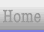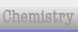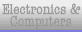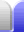# Expanding

Expanding is the opposite of factorising. Expanding is removing the brackets in an expression with brackets.

We'll work through this example starting from the front. The  3x2 is multiplied by each of the terms inside the first set of brackets.

 3x2(4 + x2)(2 + x)(2 - x) = (12x2 + 3x4)(2 + x)(2 - x)

That was easy enough. Note that terms with different powers of x cannot be combined with each other because they are not "like" terms.

To do the next two sets of brackets we need to multiply each term in the first set of brackets by each term in the second set of brackets. To help us keep track of which ones we've done we use the acronym FOIL - first, outer, inner, last. In other words, we multiply the first terms together, then the outer terms, and so on.

 3x2(4 + x2)(2 + x)(2 - x) = (12x2 + 3x4)(2 + x)(2 - x) = (24x2 + 12x3 + 6x4 + 3x5)(2 - x)

At this point I'm going to break up the second set of brackets (a partial expansion) then expand each one. Note that the minus sign of the "- x" applies to the whole of the last set of brackets. When removing the brackets it changes the sign of every term in the brackets.

 (24x2 + 12x3 + 6x4 + 3x5)(2 - x) = 2(24x2 + 12x3 + 6x4 + 3x5) - x(24x2 + 12x3 + 6x4 + 3x5) = 48x2 + 24x3 + 12x4 + 6x5 - (24x3 + 12x4 + 6x5 + 3x6) = 48x2 + 24x3 + 12x4 + 6x5 - 24x3 - 12x4 - 6x5 - 3x6

From there, there's just one more step to get the answer - simplify.Home Astronomy Chemistry Electronics Mathematics Physics Field Trips Turn on javascript for email link